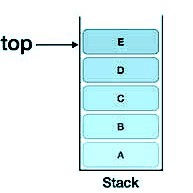Given an infix expression in the form of a string. Convert this infix expression to postfix expression.

Infix expression is an expression of the form a op b. When an operator is in-between every pair of operands.

Postfix expression is an expression of the form a b op. When an operator is followed for every pair of operands.Input:

The first line of input contains an integer T denoting the number of test cases. The next T lines contain an infix expression. The expression contains all characters and ^,*,/,+,-.

Output:

For each test case, in a new line, output the infix expression to postfix expression.

This problem has been previously asked in Amazon, Paytm, and Samsung.

We will be using the Stack Data Structure to solve the given problem.

A small recap — You can think of a Stack a pile of objects and we can basically perform two functions on it, push and pop. The push operation adds an object to the top of the stack and the pop operation deletes an item from the top of the stack.

Coming to the problem, we have to convert infix expression to postfix expression. The algorithm for this is as follows -

1. Read the input expression from the beginning.
2. If the current read character is ‘(‘, push it into the stack.
3. If the current read character is ‘)’, start popping characters from the stack, till we find ‘(‘, and pop this too.
4. If the current read character is ‘^’, ‘*’, ‘/’, ‘+’ or ‘-’ (an operator), then perform the following operations -
5. If the top of the stack == ‘(‘, push the operator into the stack,
6. Else if the precedence of the current operator is greater than the precedence of the operator at the top of the stack, then push the current operator.
7. Else if the precedence of the current operator is less than the precedence of the operator at the top of the stack, then start popping the operators from the stack till you find an operator of the precedence which is greater than or equal to that of the current operator or till we find ‘(‘ or till the stack gets empty.
8. Repeat Step 2 to Step 4 until the last character of the input expression is read.
9. After the complete input expression is read pop the remaining operators from the stack.

The below-given code is in the C programming language.

```#include<stdio.h>
#include<string.h>

int top = -1;

void push(char *arr, char ch){
top = top + 1;
arr[top] = ch;
}

void pop(char *arr){
if(arr[top] != '('){
printf("%c", arr[top]);
}
top = top - 1;
}

int precedence(char ch){
if(ch == '^')
return 3;
else if(ch == '*' || ch == '/')
return 2;
else if(ch == '+' || ch == '-')
return 1;
else
return -1;
}

void main(){
char str, arr, ch;
int a, n, t;
scanf("%d", &t);
while(t != 0){
scanf("%s", str);
n = strlen(str);
for(a = 0 ; a < n ; a++){
ch = str[a];
if(ch == '('){
push(arr, ch);
}
else if(ch == ')'){
while(arr[top] != '('){
pop(arr);
}
pop(arr);
}
else if(ch == '^' || ch == '*' || ch == '/' || ch == '+' || ch == '-'){
if(arr[top] == '('){
push(arr, ch);
}
else if(precedence(ch) > precedence(arr[top]) || top == -1){
push(arr, ch);
}
else{
while(precedence(ch) <= precedence(arr[top])){
if(arr[top] == '(' || top == -1){
break;
}
else{
pop(arr);
}
}
push(arr, ch);
}
}
else{
printf("%c", ch);
}
}

while(top != -1){
pop(arr);
}
printf("\n");
t--;
}
}
```